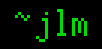## 21-Apr-2012

### Fun with metafunctions

Filed under: math — jlm @ 23:14

In mathematics, a function is a mapping from one set (the domain) onto another (not necessarily distinct) set (the range). Now, you can cause all kinds of weirdness if you let elements of these sets be functions themselves. Like, you can define a function k from integers to functions from integers to integers, which are the “constant” functions of that integer: if j = k(x) for some x in Z, then j(y) = x for all y in Z. j is a function which carries the x inside it, waiting to be applied to an argument, which is ignored and now the hidden x emerges. Written more densely, k(x)(y) = x for all x, y in Z.

But mathematics doesn’t allow you to do what’d be the most fun: Calling a function on itself. This is because you’d have to include a function in its own domain, and you get to an infinite regress, because the formal definition of the function includes specifying its domain which contains the function whose formal definition includes its domain … and you can’t build such a thing in set theory.

Okay, suppose I try to get around this problem by introducing a set Σ containing non-function elements which maps 1:1 to the functions from Σ to the integers Z. This can’t work because Σ has to be “bigger” than itself. So, suppose Σ only maps to a few of these functions. This seems like it should do, and I can just declare that any several functions I’m interested in have mappings to Σ, and functions obtained by Cantor diagonalization simply won’t. Let a be the function that maps (some of the functions from Σ to Z) onto Σ and b be its inverse.

Neither a nor b is a function from Σ to Z, so I can’t apply a(a) or b(b), so my attempts at self-applying need to be more subtle.
Let’s define a function from Σ to Z, and declare it to be a element of a’s domain:
f(ξ) = b(ξ)(ξ) + 1
here ξ is a element of Σ, so b(ξ) is a function from Σ to Z, and b(ξ)(ξ) an integer, so it seems to work out.

I declared f to be in a’s domain, so a(f) exists and is an element of Σ, let’s call it θ.
Now I can finally achieve something close to application-on-itself with f(a(f)) = f(θ).
What can we say about this? Well, its value depends on b(θ)(θ).
But b is the inverse of a, so b(θ) = f, and so b(θ)(θ) = f(θ). But we defined f so that f(ξ) and b(ξ)(ξ) could never have the same value!

See what fun functions of functions returning functions are.